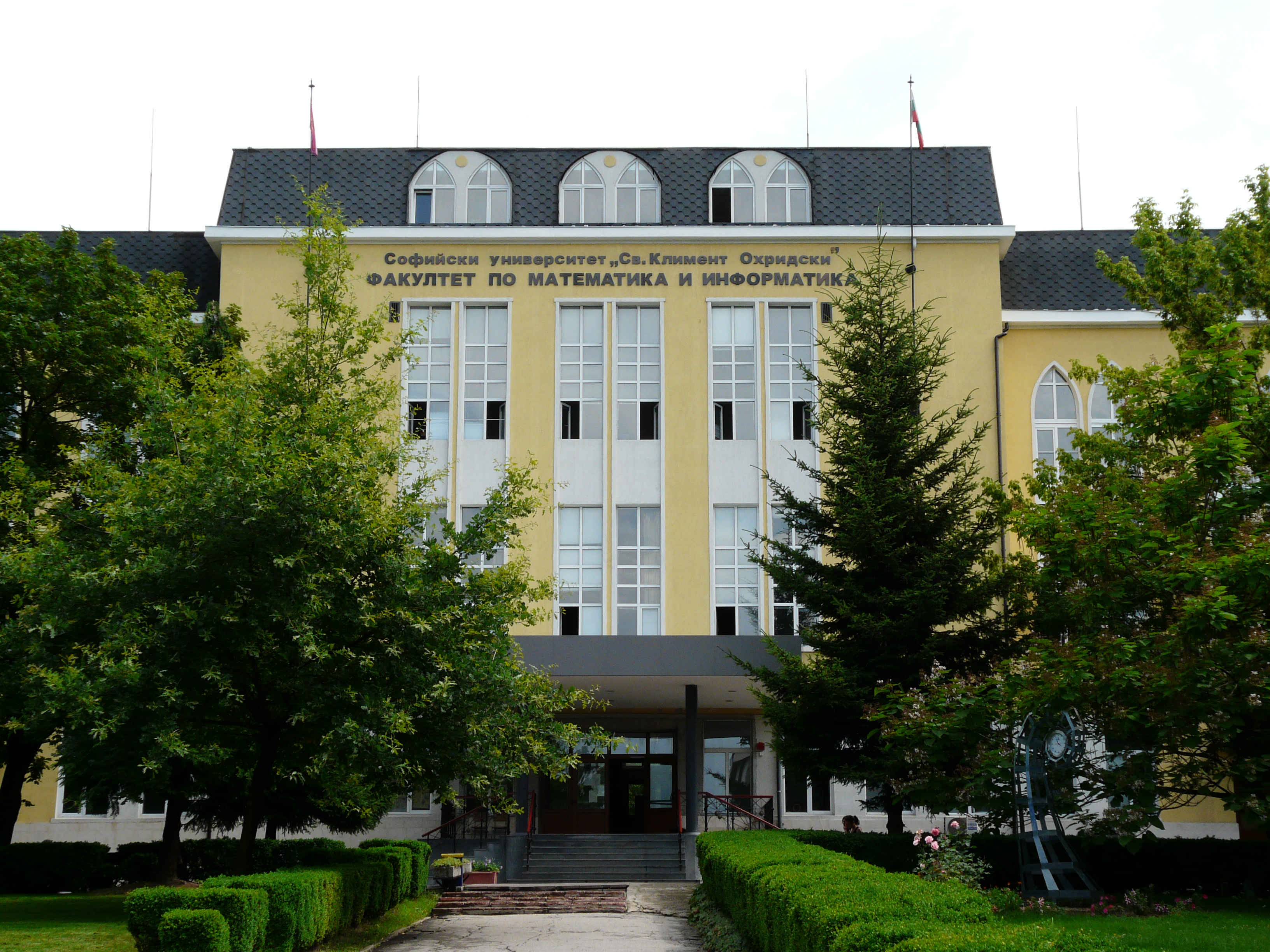## A successful direction of studies of the students at the MSc program “Computational Mathematics and Mathematical Modelling” at the Faculty of Mathematics and Informatics, Sofia UniversityThe MSc Program “Computational Mathematics and Mathematical Modelling” at the Faculty of Mathematics and Informatics at Sofia University is certified by ECMI as a Model Msc Program in Technomathematics. It gives a broad choice of courses in Mathematical Modelling, Numerical methods and fundamental mathematical disciplines, needed for working in the […]